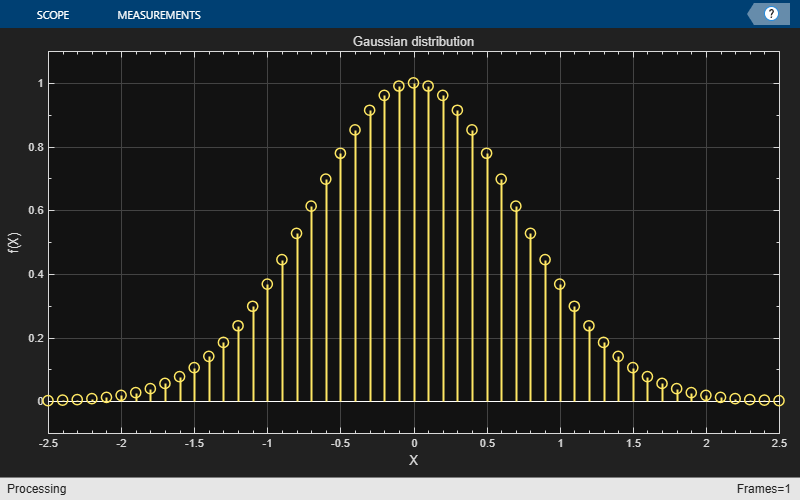# printToFigure

Print scope window to MATLAB figure

Since R2023b

## Syntax

``printToFigure(scope)``
``fig = printToFigure(scope)``
``fig = printToFigure(scope,Visible=flag)``

## Description

``` `printToFigure(scope)` prints the display window of the `scope` object to a new MATLAB® figure. The figure is visible by default.```

example

``` `fig = printToFigure(scope)` prints the display window of the `scope` object to a new MATLAB figure and returns the handle to the figure.```

example

``` `fig = printToFigure(scope,Visible=flag)` shows or hides the MATLAB figure.```

## Examples

collapse all

Use the `printToFigure` function to print the `spectrumAnalyzer` object display window to a new MATLAB® figure.

Generate a chirp signal and use the `spectrumAnalyzer` object to display the spectrum of the chirp.

```chirp = dsp.Chirp(SweepDirection="Bidirectional", ... TargetFrequency=2000, ... InitialFrequency=0,... TargetTime=400, ... SweepTime=400, ... SamplesPerFrame=1024, ... SampleRate=4000); scope = spectrumAnalyzer(AveragingMethod="exponential",... ForgettingFactor=0,SampleRate=4000); scope(chirp());```Print the display of the chirp spectrum to a new MATLAB figure. The function returns a handle to the figure.

`scopeFig = printToFigure(scope);`The handle to the figure `scopeFig` lets you modify the appearance and the behavior of the figure window.

Specify a figure name and change the size of the figure to 400-by-250 pixels.

```scopeFig.Name="Spectrum of Chirp Signal"; scopeFig.NumberTitle="off"; scopeFig.Position=[1 1 400 250];```When printing to figure, you can make the figure invisible by setting the` Visible` argument to `false`.

`scopeFig = printToFigure(scope,Visible=false);`

Use the `printToFigure` function to print the `dsp.ArrayPlot` object display window to a new MATLAB® figure.

Create a `dsp.ArrayPlot` object.

`scope=dsp.ArrayPlot;`

Set `ArrayPlot` properties to display a Gaussian distribution.

```scope.YLimits = [-0.1 1.1]; scope.XOffset = -2.5; scope.SampleIncrement = 0.1; scope.Title = "Gaussian distribution"; scope.XLabel = "X"; scope.YLabel = "f(X)";```

Plot the Gaussian distribution.

`scope(exp(-(-2.5:.1:2.5) .* (-2.5:.1:2.5))');`Print the display of the Gaussian distribution to a new MATLAB figure. The function returns a handle to the figure.

`scopeFig = printToFigure(scope);`The handle to the figure `scopeFig` lets you modify the appearance and the behavior of the figure window.

Specify a figure name and change the size of the figure to 400-by-250 pixels.

```scopeFig.Name="Gaussian Distribution"; scopeFig.NumberTitle="off"; scopeFig.Position=[1 1 400 250];```When printing to figure, you can make the figure invisible by setting the `Visible` argument to `false`.

`scopeFig = printToFigure(scope,Visible=false);`

Use the `printToFigure` function to print the `timescope` object display window to a new MATLAB® figure.

View a sine wave on the Time Scope.

```f = 100; fs = 1000; swv = sin(2.*pi.*f.*(0:1/fs:1-1/fs)).'; scope = timescope(SampleRate=fs,... TimeSpanSource="property", ... TimeSpan=1); scope(swv);```Print the display of the sine wave to a new MATLAB figure. The function returns a handle to the figure.

`scopeFig = printToFigure(scope);`The handle to the figure `scopeFig` lets you modify the appearance and the behavior of the figure window.

Specify a figure name and change the size of the figure to 400-by-250 pixels.

```scopeFig.Name="Sine Wave Signal"; scopeFig.NumberTitle="off"; scopeFig.Position=[1 1 400 250];```When printing to figure, you can make the figure invisible by setting the `Visible` argument to `false`.

`scopeFig = printToFigure(scope,Visible=false);`

Use the `printToFigure` function to print the `dsp.DynamicFilterVisualizer` object display window to a new MATLAB® figure.

Create a `dsp.DynamicFilterVisualizer` object.

`dfv = dsp.DynamicFilterVisualizer(YLimits=[-120 10]);`

Design FIR filter with varying cutoff frequencies ranging from 0.1 to 0.5. Plot the magnitude response of the filter using the Dynamic Filter Visualizer.

```for k = 0.1:0.001:0.5 b = fir1(90, k); dfv(b,1); end ```Print the display of the magnitude response to a new MATLAB figure. The function returns a handle to the figure.

`scopeFig = printToFigure(dfv);`The handle to the figure `scopeFig` lets you modify the appearance and the behavior of the figure window.

Specify a figure name and change the size of the figure to 400-by-250 pixels.

```scopeFig.Name="Magnitude Response of FIR Filter"; scopeFig.NumberTitle="off"; scopeFig.Position=[1 1 400 250];```When printing to figure, you can make the figure invisible by setting the `Visible` argument to `false`.

`scopeFig = printToFigure(dfv,Visible=false);`

## Input Arguments

collapse all

Scope object whose display the function prints to a MATLAB figure, specified as one of the following:

Flag to show the MATLAB figure, specified as `true` or `false`.

Data Types: `logical`

## Output Arguments

collapse all

Handle to the MATLAB figure, returned as a `Figure` object. For more information on the properties of this object, see Figure Properties.

## Version History

Introduced in R2023b